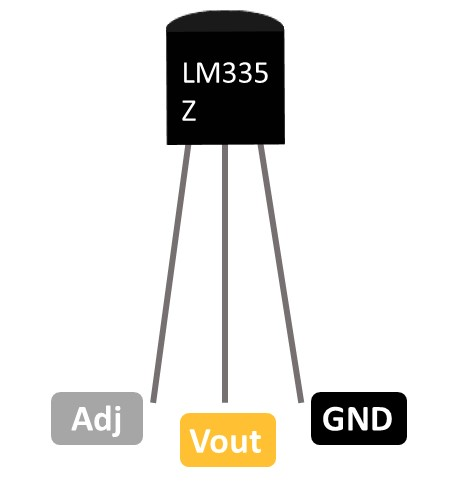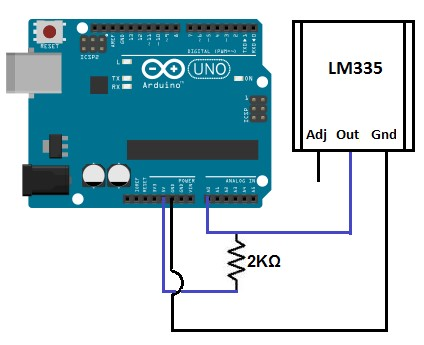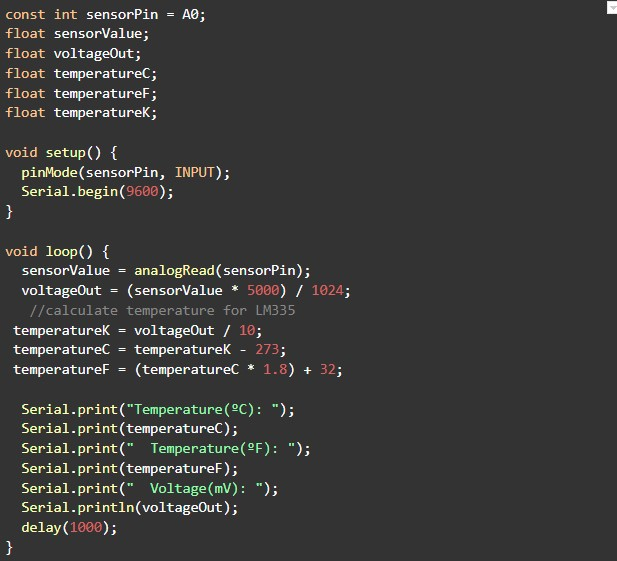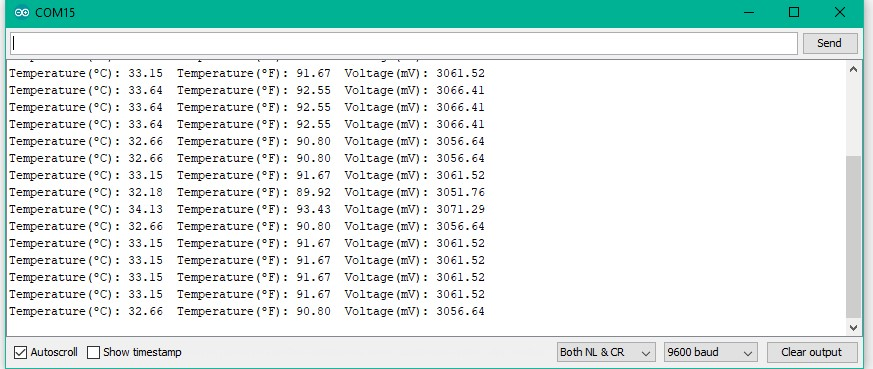# Evelta LM335Z Analog Temperature Sensor Probe

No reviews yet
Evelta SKU:
011-EVE-LM335Z-PR
Manufacturer Part Number:
EVE-LM335Z-PR
₹230.10 inc. GST
₹195.00 ex. GST
Request a quote for higher quantities

## Description

The LM335 is an easy to use, relatively precise temperature sensor. It is great for things like temperature loggers and temperature controllers. This operates as a 2-terminal Zener and the breakdown voltage is directly proportional to the absolute temperature at 10mV/ K. The circuit has a dynamic impedance of less than 1 Ohm and operates within a range of current from 450uA to 5mA without alteration of its characteristicsThis sensor is much easier to use than thermocouples, because it does not require any additional interface circuitry. It is also easier than thermistors or other resistive temperature sensors, since it requires no calibration, and has a linear response.

Key Features
• Directly calibrated in K
• 1C initial accuracy
• Operates from 450uA to 5mA
• Less than 1Ohm dynamic impedance
• Temperature Sensing Range -40 ~ 100C
Resources

#### LM335Z Pinout#### LM335 Temperature Sensor with Arduino

Components Required
1. An LM335 sensor
3. An arduino board
4. Some jumper wires
5. A 2k resistor

#### LM335 Wiring with Arduino Connection DiagramPin Connections
• Pin 1 is the Adjustable Pin (Adj). This allows us to calibrate the temperature sensor if more precise temperature is required. It is not needed for testing.
• Pin 2 is the output pin. Attach this pin to analog pin A0 of the arduino board and connect the 2KOhm resistor to the 5V pin of the arduino.
• Pin 3 is the ground pin and it connects to the ground (GND) terminal of the arduino.

#### Arduino Code for LM335 Temperature Sensor Circuit#### Code OutputBefore we can get a Kevlin reading of the temperature, the analog output voltage must first be read. This will be the raw value divided by 1024 times 5000. It is divided by 1024 because a span of 1024 occupies 5V. We get the ratio of the raw value to the full span of 1024 and then multiply it by 5000 to get the millivolt value. Since the output pin can give out a maximum of 5 volts (1024), 1024 represents the full possible range it can give out. The raw voltage over this 1024 (value) therefore represents the ratio of how much power the output pin is outputting against this full range. Once we have this ratio, we then multiply it by 5000 to give the millivolt value. This is because there is 5000 millvolts in 5 volts.
Once this analog voltage in millivolts is calculated, we then can find the temperature in kelvin by the equation: (millivolts/10).
To get the equivalent temperature in Celsius, we subtract the Kelvin value by 273. Once we obtain this Celsius value, we can convert into Fahrenheit with the following equation: (celsius * 1.8 +32). At the end of this program, we put a delay of 1000ms to take the temperature reading every second.

## Extra Information

Brand:
Evelta
Max Operating Temperature:
100 C
Max Supply Voltage:
40 V
Max Supply Voltage (DC):
40 V
Min Operating Temperature:
-40 C
Min Supply Voltage:
5 V
Operating Supply Current:
1 mA
Output Current:
450 uA
Output Type:
Analog, Analog Voltage
Probe Length:
2 Inch
Cable Length:
1 Meter

## Warranty Information

All the products supplied by Evelta are genuine and original. We offer 14 days replacement warranty in case of manufacturing defects. For more details, please visit our cancellation and returns page.